﻿ JavaScript内置对象教程 – 码云笔记

# JavaScript内置对象教程

102℃

[隐藏]

## 什么是对象

JavaScript 中的所有事物都是对象，如:字符串、数值、数组、函数等，每个对象带有属性和方法。

JavaScript 提供多个内建对象，比如 String、Date、Array 等等，使用对象前先定义，如下使用数组对象：

``````var objectName =new Array();//使用new关键字定义对象

var objectName =[];``````

``objectName.propertyName``

``````var myarray=new Array(6);//定义数组对象
var myl=myarray.length;//访问数组长度length属性``````

``objectName.methodName()``

``````var mystr="Hello world!";//创建一个字符串
var request=mystr.toUpperCase(); //使用字符串对象方法``````

## Date 日期对象

``var Udate=new Date();``

``````var d = new Date(2012, 10, 1);  //2012年10月1日
var d = new Date('Oct 1, 2012'); //2012年10月1日``````

Date对象中处理时间和日期的常用方法：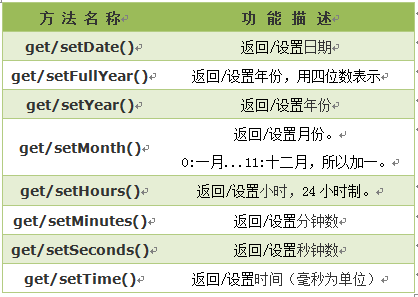## 返回/设置年份方法

get/setFullYear() 返回/设置年份，用四位数表示。

``````var mydate=new Date();//当前时间2014年3月6日
document.write(mydate+"<br>");//输出当前时间
document.write(mydate.getFullYear()+"<br>");//输出当前年份
mydate.setFullYear(81); //设置年份
document.write(mydate+"<br>"); //输出年份被设定为 0081年。``````

``````Thu Mar 06 2014 10:57:47 GMT+0800
2014
Thu Mar 06 0081 10:57:47 GMT+0800``````

1.结果格式依次为：星期、月、日、年、时、分、秒、时区。(火狐浏览器)

2. 不同浏览器，时间格式有差异。

## 返回星期方法

getDay() 返回星期，返回的是0-6的数字，0 表示星期天。如果要返回相对应“星期”，通过数组完成，代码如下:

``````<script type="text/javascript">
var mydate=new Date();//定义日期对象
var weekday=["星期日","星期一","星期二","星期三","星期四","星期五","星期六"];
//定义数组对象,给每个数组项赋值
var mynum=mydate.getDay();//返回值存储在变量mynum中
document.write(mydate.getDay());//输出getDay()获取值
document.write("今天是："+ weekday[mynum]);//输出星期几
</script>``````

``````5

## 返回/设置时间方法

get/setTime() 返回/设置时间，单位毫秒数，计算从 1970 年 1 月 1 日零时到日期对象所指的日期的毫秒数。

``````<script type="text/javascript">
var mydate=new Date();
document.write("当前时间："+mydate+"<br>");
mydate.setTime(mydate.getTime() + 60 * 60 * 1000);
document.write("推迟一小时时间：" + mydate);
</script>``````

``````当前时间：Thu Mar 6 11:46:27 UTC+0800 2014

1. 一小时 60 分，一分 60 秒，一秒 1000 毫秒

2. 时间推迟 1 小时,就是: “x.setTime(x.getTime() + 60 * 60 * 1000);”

## String 字符串对象

``var mystr = "I love JavaScript!"``

stringObject.length; 返回该字符串的长度

``````var mystr="Hello World!";
var myl=mystr.length;``````

``````var mystr="Hello world!";
var mynum=mystr.toUpperCase();``````

## 返回指定位置的字符

charAt() 方法可返回指定位置的字符。返回的字符是长度为 1 的字符串。

``stringObject.charAt(index)``

index 必需。表示字符串中某个位置的数字，即字符在字符串中的下标。

1.字符串中第一个字符的下标是 0。最后一个字符的下标为字符串长度减一（string.length-1）。

2.如果参数 index 不在 0 与 string.length-1 之间，该方法将返回一个空字符串。

``````<script type="text/javascript">
var mystr="I love JavaScript!"
document.write(mystr.charAt(2));
</script>``````

``l``

## 返回指定的字符串首次出现的位置

indexOf() 方法可返回某个指定的字符串值在字符串中首次出现的位置。

``stringObject.indexOf(substring, startpos)``

substring 必需。规定需检索的字符串值。
startpos 可选的整数参数。规定在字符串中开始检索的位置。它的合法取值是 0 到 stringObject.length – 1。如省略该参数，则将从字符串的首字符开始检索。

1.该方法将从头到尾地检索字符串 stringObject，看它是否含有子串 substring。

2.可选参数，从stringObject的startpos位置开始查找substring，如果没有此参数将从stringObject的开始位置查找。

3.如果找到一个 substring，则返回 substring 的第一次出现的位置。stringObject 中的字符位置是从 0 开始的。

1.indexOf() 方法区分大小写。

2.如果要检索的字符串值没有出现，则该方法返回 -1。

``````<script type="text/javascript">
var str="I love JavaScript!"
document.write(str.indexOf("I") + "<br />");
document.write(str.indexOf("v") + "<br />");
document.write(str.indexOf("v",8));
</script>``````

``````0
4
9``````

## 字符串分割split()

split() 方法将字符串分割为字符串数组，并返回此数组。

``stringObject.split(separator,limit)``

separator 必需。从该参数指定的地方分割stringObject。
limit 可选的参数，分割次数，如设置该参数，返回的子串不会多于这个参数指定的数组，如果无此参数为不限制次数。

``````var mystr = "www.mybj123.com";
document.write(mystr.split(".")+"<br>");
document.write(mystr.split(".", 2)+"<br>");``````

``````www,mybj123,com
www,mybj123``````

``````document.write(mystr.split("")+"<br>");
document.write(mystr.split("", 5));``````

``````w,w,w,.,m,y,b,j,1,2,3,.,c,o,m
w,w,w,.,m``````

## 提取字符串substring()

substring() 方法用于提取字符串中介于两个指定下标之间的字符。

``stringObject.substring(startPos,stopPos)``

startPos 必需。一个非负的整数，开始位置。
stopPos 可选。一个非负的整数，结束位置。如果省略该参数，那么返回的子串会一直到字符串对象的结尾。

1. 返回的内容是从 start开始(包含start位置的字符)到 stop-1 处的所有字符，其长度为 stop 减start。

2. 如果参数 start 与 stop 相等，那么该方法返回的就是一个空串（即长度为 0 的字符串）。

3. 如果 start 比 stop 大，那么该方法在提取子串之前会先交换这两个参数。

``````<script type="text/javascript">
var mystr="I love JavaScript";
document.write(mystr.substring(7));
document.write(mystr.substring(2,6));
</script>``````

``````JavaScript
love``````

## 提取指定数目的字符substr()

substr() 方法从字符串中提取从 startPos位置开始的指定数目的字符串。

``stringObject.substr(startPos,length)``

startPos 必需。要提取的子串的起始位置。必须是数值。
length 可选。提取字符串的长度。如果省略，返回从stringObject的开始位置startPos到stringObject的结尾的字符。

``````<script type="text/javascript">
var mystr="I love JavaScript!";
document.write(mystr.substr(7));
document.write(mystr.substr(2,4));
</script>``````

``````JavaScript!
love``````

## Math对象

Math对象，提供对数据的数学计算。

``````<script type="text/javascript">
var mypi=Math.PI;
var myabs=Math.abs(-15);
document.write(mypi);
document.write(myabs);
</script>``````

``````3.141592653589793
15``````

Math 对象属性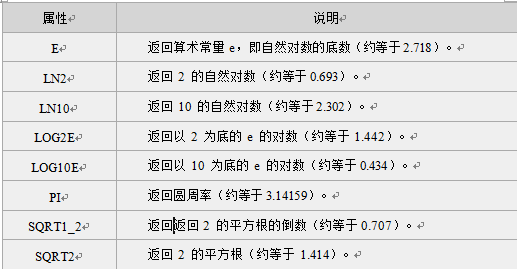Math 对象方法

##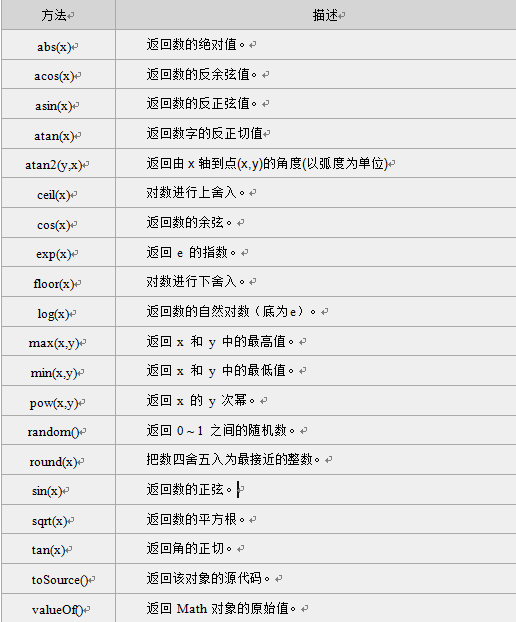向上取整ceil()

ceil() 方法可对一个数进行向上取整。

``Math.ceil(x)``

x 必需。必须是一个数值。

``````<script type="text/javascript">
document.write(Math.ceil(0.8) + "<br />")
document.write(Math.ceil(6.3) + "<br />")
document.write(Math.ceil(5) + "<br />")
document.write(Math.ceil(3.5) + "<br />")
document.write(Math.ceil(-5.1) + "<br />")
document.write(Math.ceil(-5.9))
</script>``````

``````1
7
5
4
-5
-5``````

## 向下取整floor()

floor() 方法可对一个数进行向下取整。

``Math.floor(x)``

x 必需。必须是一个数值。

``````<script type="text/javascript">
document.write(Math.floor(0.8)+ "<br>")
document.write(Math.floor(6.3)+ "<br>")
document.write(Math.floor(5)+ "<br>")
document.write(Math.floor(3.5)+ "<br>")
document.write(Math.floor(-5.1)+ "<br>")
document.write(Math.floor(-5.9))
</script>``````

``````0
6
5
3
-6
-6``````

## 四舍五入round()

round() 方法可把一个数字四舍五入为最接近的整数。

``Math.round(x)``

x 必需。必须是数字。

1. 返回与 x 最接近的整数。

2. 对于 0.5，该方法将进行上舍入。(5.5 将舍入为 6)

3. 如果 x 与两侧整数同等接近，则结果接近 +∞方向的数字值 。(如 -5.5 将舍入为 -5; -5.52 将舍入为 -6),如下图: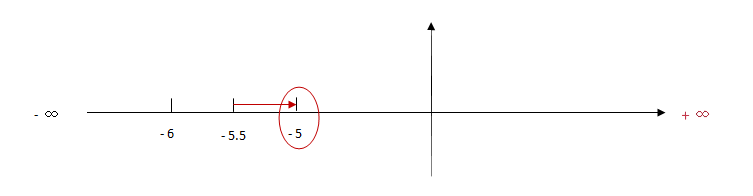``````<script type="text/javascript">
document.write(Math.round(1.6)+ "<br>");
document.write(Math.round(2.5)+ "<br>");
document.write(Math.round(0.49)+ "<br>");
document.write(Math.round(-6.4)+ "<br>");
document.write(Math.round(-6.6));
</script>``````

``````2
3
0
-6
-7``````

## 随机数 random()

random() 方法可返回介于 0 ~ 1（大于或等于 0 但小于 1 )之间的一个随机数。

``Math.random();``

``````<script type="text/javascript">
document.write(Math.random());
</script>``````

``0.190305486195328``

``````<script type="text/javascript">
document.write((Math.random())*10);
</script>``````

``8.72153625893887``

## Array 数组对象

1. 定义了一个空数组:

``var  数组名= new Array();``

2. 定义时指定有n个空元素的数组：

``var 数组名 =new Array(n);``

3.定义数组的时候，直接初始化数据：

``var  数组名 = [<元素1>, <元素2>, <元素3>...];``

``var myArray = [2, 8, 6]; ``

``数组名[下标] = 值;``

length 用法：<数组对象>.length；返回：数组的长度，即数组里有多少个元素。它等于数组里最后一个元素的下标加一。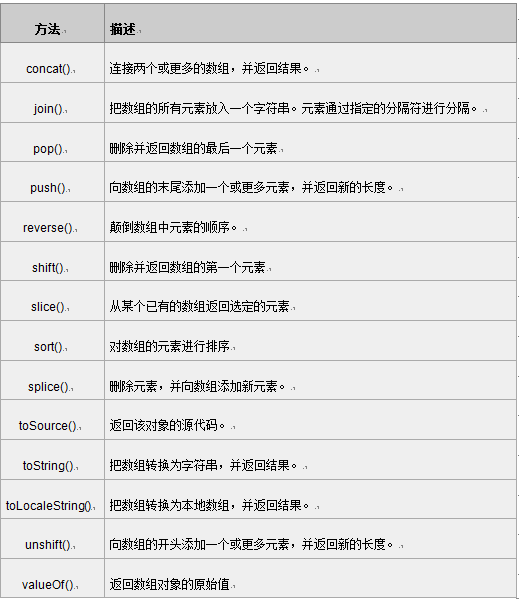## 数组连接concat()

concat() 方法用于连接两个或多个数组。此方法返回一个新数组，不改变原来的数组。

``arrayObject.concat(array1,array2,...,arrayN)``

array1 要连接的第一个数组。

arrayN 第N额数组

``````<script type="text/javascript">
var mya = new Array(3);
mya = "1";
mya = "2";
mya = "3";
document.write(mya.concat(4,5)+"<br>");
document.write(mya);
</script>``````

``````1,2,3,4,5
1,2,3``````

``````<script type="text/javascript">
var mya1= new Array("hello!")
var mya2= new Array("I","love");
var mya3= new Array("JavaScript","!");
var mya4=mya1.concat(mya2,mya3);
document.write(mya4);
</script>``````

``hello!,I,love,JavaScript,!``

## 指定分隔符连接数组元素join()

join()方法用于把数组中的所有元素放入一个字符串。元素是通过指定的分隔符进行分隔的。

``arrayObject.join(分隔符)``

separator 可选。指定要使用的分割符。如果省略该参数，则使用逗号作为分割符。

``````<script type="text/javascript">
var myarr = new Array(3);
myarr = "I";
myarr = "love";
myarr = "JavaScript";
document.write(myarr.join());
</script>``````

``I,love,JavaScript``

``````<script type="text/javascript">
var myarr = new Array(3)
myarr = "I";
myarr = "love";
myarr = "JavaScript";
document.write(myarr.join("."));
</script>``````

``I.love.JavaScript``

## 颠倒数组元素顺序reverse()

reverse() 方法用于颠倒数组中元素的顺序。

``arrayObject.reverse()``

``````<script type="text/javascript">
var myarr = new Array(3)
myarr = "1"
myarr = "2"
myarr = "3"
document.write(myarr + "<br />")
document.write(myarr.reverse())
</script>``````

``````1,2,3
3,2,1``````

## 选定元素slice()

slice() 方法可从已有的数组中返回选定的元素。

``arrayObject.slice(start,end)``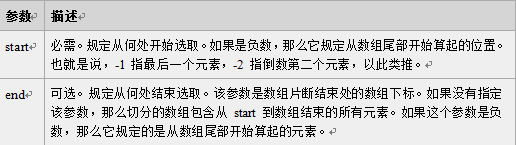1.返回一个新的数组，包含从 start 到 end （不包括该元素）的 arrayObject 中的元素。

2. 该方法并不会修改数组，而是返回一个子数组。

1. 可使用负值从数组的尾部选取元素。

2.如果 end 未被规定，那么 slice() 方法会选取从 start 到数组结尾的所有元素。

3. String.slice() 与 Array.slice() 相似。

``````<script type="text/javascript">
var myarr = new Array(1,2,3,4,5,6);
document.write(myarr + "<br>");
document.write(myarr.slice(2,4) + "<br>");
document.write(myarr);
</script>``````

``````1,2,3,4,5,6
3,4
1,2,3,4,5,6``````

## 数组排序sort()

sort()方法使数组中的元素按照一定的顺序排列。

``arrayObject.sort(方法函数)``

1.如果不指定<方法函数>，则按unicode码顺序排列。

2.如果指定<方法函数>，则按<方法函数>所指定的排序方法排序。

``myArray.sort(sortMethod);``

1.使用sort()将数组进行排序，代码如下：

``````<script type="text/javascript">
var myarr1 = new Array("Hello","John","love","JavaScript");
var myarr2 = new Array("80","16","50","6","100","1");
document.write(myarr1.sort()+"<br>");
document.write(myarr2.sort());
</script>``````

``````Hello,JavaScript,John,love
1,100,16,50,6,80``````

2.如要实现这一点，就必须使用一个排序函数，代码如下：

``````<script type="text/javascript">
function sortNum(a,b) {
return a - b;
//升序，如降序，把“a - b”该成“b - a”
}
var myarr = new Array("80","16","50","6","100","1");
document.write(myarr + "<br>");
document.write(myarr.sort(sortNum));
</script>``````

``````80,16,50,6,100,1
1,6,16,50,80,100``````

## 编程练习

``XXXX年XX月X日 星期X--班级总分为:81``

1、显示打印的日期。 格式为类似“XXXX年XX月XX日 星期X” 的当前的时间。

2、计算出该班级的平均分（保留整数）。

“小明:87; 小花:81; 小红:97; 小天:76;小张:74;小小:94;小西:90;小伍:76;小迪:64;小曼:76″

``````<!DOCTYPE  HTML>
<html >
<meta http-equiv="Content-Type" content="text/html; charset=utf-8" />
<title>系好安全带,准备启航</title>

<script type="text/javascript">

//通过javascript的日期对象来得到当前的日期，并输出。
var mydate = new Date();
var weekday = ["星期日", "星期一", "星期二", "星期三", "星期四", "星期五", "星期六"];
document.write(mydate.getFullYear() + "年" +
(mydate.getMonth() + 1) + "月" +
mydate.getDay() + "日" + " " +
weekday[mydate.getDay()]
);

//成绩是一长窜的字符串不好处理，找规律后分割放到数组里更好操作哦
var scoreStr = "小明:87;小花:81;小红:97;小天:76;小张:74;小小:94;小西:90;小伍:76;小迪:64;小曼:76";
var scoreArr = scoreStr.split(";");

//document.write(scoreArr);
//从数组中将成绩撮出来，然后求和取整，并输出。

var score = new Array();
var sum = 0;
for (var i = 0; i < scoreArr.length; i ++) {
sum += parseInt(scoreArr[i].substr(scoreArr[i].indexOf(":")+1));
//document.write("<br>" + sum);//test
}
document.write("--班级总分为:" + sum);
document.write(",班级平均分为(保留整数):" + Math.round(sum/scoreArr.length))

</script>